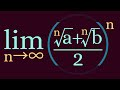## Stream: Beginner Questions

### Topic: Limit proof with real power

####Mark Wassell (Jan 05 2021 at 08:36):

Hi,

I would like to prove that for $a>0$ :

$\lim\limits_{x \rightarrow 0^+} a^x = 1$

In Isabelle, this might look something like:

lemma
fixes a::real
assumes "a > 0"
shows "((λx. a ^ x) ⤏ 1) F"


what filter should I used for 'tends to zero from above' and is there a real power function that makes x real? In the above currently, Isabelle is inferring nat as the type for x.

This is part of a personal study to see how easy it is to do some basic limit problems in a nice and accessible way. Limit problems such as this one on Youtube.Cheers

Mark

####Manuel Eberl (Jan 05 2021 at 11:24):

Funny you should ask, if you import HOL-Real_Asymp.Real_Asymp, this goes through with using assms by real_asymp. :smile:

####Manuel Eberl (Jan 05 2021 at 11:28):

And as for your other questions, x powr y is the power function on real numbers (caveat: 0 ^ y = 0 for any y and it's undefined for x < 0) or complex numbers (same caveat for x = 0, branch cut for negative real y as usual).

The F in your case should simply be at_right 0. This is an abbreviation for at 0 within {0<..}, and at x within A is basically the neighbourhood filter nhds x restricted to A - {x}, i.e. you can approach x from any ‘path’ within A-{x}.

You can even prove something stronger, namely filterlim (λx. a powr x) (at_right 1) (at_right 0).

####Manuel Eberl (Jan 05 2021 at 11:49):

Correction: You need a case distinction over a < 1, a = 1 and a > 1 though because real_asymp works by computing series expansions and these look a bit different in each of these cases: without the case distinction, real_asymp will tell you that it was unable to determine the sign of ln a if you run it with real_asymp (verbose).

There is also the diagnostic tool real_limit with which you can find the limit of an expression:

context
fixes a b :: real
assumes a: "a > 0" "a < 1" and b: "b > 1"
begin

real_limit "λx. a powr x"
limit: "at_right 0" facts: a

real_limit "λx. b powr x"
limit: "at_right 0" facts: b


For completeness, here the more detailed variant where you also show from where it approaches:

lemma "a ∈ {0<..<1} ⟹ filterlim (λx::real. a powr x) (at_left 1) (at_right 0)"
"a > 1 ⟹ filterlim (λx::real. a powr x) (at_right 1) (at_right 0)"
by real_asymp+


####Manuel Eberl (Jan 05 2021 at 11:55):

The video you posted shows the example $\lim\limits_{n\to\infty} \left(\frac{\sqrt[n]{a}+\sqrt[n]{b}}{2}\right)^n$. The problem with that is that for technical reasons, real_asymp currently does not support root n x where n is not a constant. However, you can prove a more general version like this:

lemma
fixes a b :: real
assumes "a > 0" "b > 0"
shows   "((λx. ((a powr (1/x) + b powr (1/x)) / 2) powr x) ⤏ exp ((ln a + ln b) / 2)) at_top"
using assms by real_asymp


There is also documentation for real_asymp in ~~/Doc/Real_Asymp. And you can of course always ask me if you have any questions!

####Manuel Eberl (Jan 05 2021 at 12:02):

Addendum: here is a proof of your initial question without real_asymp, using the continuity of powr at 0:

lemma
fixes a::real
assumes "a > 0"
shows "((λx. a powr x) ⤏ 1) (at_right 0)"
proof -
from assms have "((λx. a powr x) ⤏ a powr 0) (nhds 0)"
by (intro tendsto_intros filterlim_ident) auto
hence "((λx. a powr x) ⤏ a powr 0) (at_right 0)"
by (rule filterlim_mono) (auto simp: at_within_le_nhds)
thus ?thesis using assms by simp
qed


####Manuel Eberl (Jan 05 2021 at 12:04):

This uses the rule tendsto_powr', which is in the tendsto_intros collection:

(f ⤏ a) F ⟹ (g ⤏ b) F ⟹ a ≠ 0 ∨ 0 < b ∧ (∀⇩F x in F. 0 ≤ f x)
⟹ ((λx. f x powr g x) ⤏ a powr b) F


These proofs get fairly tedious for more complicated limits though – which is why I made real_asymp. See this paper for a description of how it works if you're curious (but for instructions on how to use it, look at the documentation instead or ask me).

####Mark Wassell (Jan 05 2021 at 15:44):

Wonderful. Thank you.

Last updated: Dec 07 2023 at 20:16 UTC